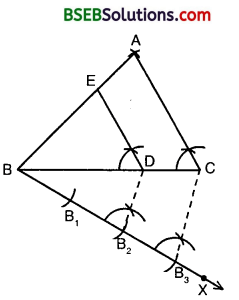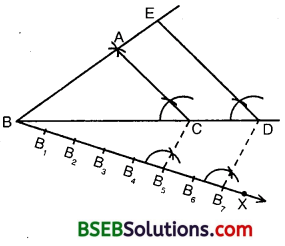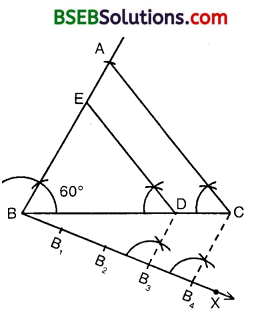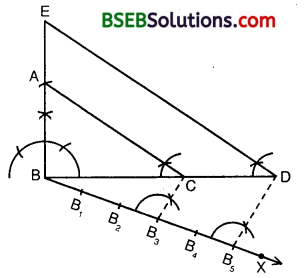# HSSlive: Plus One & Plus Two Notes & Solutions for Kerala State Board

## BSEB Class 10 Maths Chapter 11 Constructions Ex 11.1 Textbook Solutions PDF: Download Bihar Board STD 10th Maths Chapter 11 Constructions Ex 11.1 Book AnswersBSEB Class 10 Maths Chapter 11 Constructions Ex 11.1 Textbook Solutions PDF: Download Bihar Board STD 10th Maths Chapter 11 Constructions Ex 11.1 Book Answers

BSEB Class 10th Maths Chapter 11 Constructions Ex 11.1 Textbooks Solutions and answers for students are now available in pdf format. Bihar Board Class 10th Maths Chapter 11 Constructions Ex 11.1 Book answers and solutions are one of the most important study materials for any student. The Bihar Board Class 10th Maths Chapter 11 Constructions Ex 11.1 books are published by the Bihar Board Publishers. These Bihar Board Class 10th Maths Chapter 11 Constructions Ex 11.1 textbooks are prepared by a group of expert faculty members. Students can download these BSEB STD 10th Maths Chapter 11 Constructions Ex 11.1 book solutions pdf online from this page.

## Bihar Board Class 10th Maths Chapter 11 Constructions Ex 11.1 Books Solutions

 Board BSEB Materials Textbook Solutions/Guide Format DOC/PDF Class 10th Subject Maths Chapter 11 Constructions Ex 11.1 Chapters All Provider Hsslive

## How to download Bihar Board Class 10th Maths Chapter 11 Constructions Ex 11.1 Textbook Solutions Answers PDF Online?

2. Click on the Bihar Board Class 10th Maths Chapter 11 Constructions Ex 11.1 Answers.
3. Look for your Bihar Board STD 10th Maths Chapter 11 Constructions Ex 11.1 Textbooks PDF.
4. Now download or read the Bihar Board Class 10th Maths Chapter 11 Constructions Ex 11.1 Textbook Solutions for PDF Free.

Find below the list of all BSEB Class 10th Maths Chapter 11 Constructions Ex 11.1 Textbook Solutions for PDF’s for you to download and prepare for the upcoming exams:

## BSEB Bihar Board Class 10th Maths Solutions Chapter 11 Constructions Ex 11.1

Question 1.
Draw a line segment of length 7.6 cm and divide it in the ratio 5 : 8. Measure the two parts.
Solution:
Steps of Construction :1. Draw a line segment AB = 7.6 cm.

2. Draw a ray AC making any acute angle with AB, as shown in the figure.

3. On ray AC, starting from A, mark 5 + 8 = 13 equal line segments : AA1, A1A2, A2A3, A3A4, A4A5, A5A6, A6A7, A7A8, A8A9, A9A10, A10A11, A11A12 and A12A13.

4. Join A13B.

5. From A5, draw A5P || A13B, meeting AB at P.

6. Thus, P divides AB in the ratio 5 : 8.
On measuring the two parts, we find that AP = 2.9 cm and PB = 4.7 cm (approx.).

Justification :
In ∆ ABA13, PA5 || BA13.
So, 𝐴𝑃𝑃𝐵 = AA5 A5 A13 = 58 [By BPT]
Thus, AP : PB = 5 : 8

Question 2.
Construct a triangle of sides 4 cm, 5 cm and 6 cm and then a triangle similar to it whose sides are 23 of the corresponding sides of it.
Solution:
Steps of Construction :1. Draw a line segment BC = 6 cm.

2. With B as centre and radius equal to 5 cm, draw an arc.

3. With C as centre and radius equal to 4 cm, draw an arc intersecting the previously drawn arc at A.

4. Join AB and AC. Then ∆ ABC is the required triangle.

5. Below BC, make an acute angle CBX.

6. Along BX, mark off three (bigger of the numerator and denominator) points : B1, B1 and B3 such that BB1 = B1B2 = B2B3

7. Join B3C. [Note that 3 is denominator]

8. From B2 draw B2D || B3C, meeting BC at D. [Note that 2 is numerator]

9. From D, draw DE || CA, meeting BA at E. Then, ∆ EBD is the required triangle whose sides are 23 of the corresponding sides of ∆ ABC.

Justification :
Since DE || CA, therefore
∆ ABC ~ ∆ EBD
and 𝐸𝐵𝐴𝐵 = 𝐷𝐸𝐶𝐴 = 𝐵𝐷𝐵𝐶 = 23 [Since 𝐵𝐷𝐵𝐶 = 23 ,by construction]
Hence, we get the new triangle similar to the given triangle whose sides are equal to 23 of the corresponding sides of ∆ ABC.

Question 3.
Construct a triangle with sides 5 cm, 6 cm and 7 cm and then another triangle whose sides are 75 of the corresponding sides of the first triangle.
Solution:
Steps of Construction :
1. With the given data, construct ∆ ABC in which BC = 7 cm, CA = 4 cm and AB = 5 cm.

2. Below BC, make an acute angle CB1.3. Along BX, mark off seven (bigger of the numerator and denominator) points : B1, B2, B3, B4, B5, B6 and B7 such that BB1 = B1B2 = B2B3 = B3B4 = B4B5 = B5B6 = B6B7

4. Join B5C. [Note that 5 is denominator]

5. From B7, draw B7D || B5C, meeting BC produced at D. [Note that 7 is numerator]

6. From D, draw DE || CA, meeting BA produced at E. Then, ∆ EBD is the required triangle whose sides are 75 of the corresponding sides of ∆ ABC.

Justification :
Since DE || CA, therefore
∆ ABC ~ ∆ EBD and 𝐸𝐵𝐴𝐵 = 𝐷𝐸𝐶𝐴 = 𝐵𝐷𝐵𝐶 = 75
Hence, we get the new triangle similar to the given triangle whose sides are equal to 75 of the corresponding sides of ∆ ABC.

Question 4.
Construct an isosceles triangle whose base is 8 cm and altitude 4 cm and then another triangle whose sides are 112 times the corresponding sides of the isosceles triangle.
Solution:
Steps of Construction :1. Draw BC = 8 cm.

2. Construct PQ, the perpendicular bisector of line segment BC meeting BC at M.

3. Along MP, cut off MA = 4 cm.

4. Join BA and CA. Then, ∆ ABC so obtained is the required triangle.

5. Make an acute angle CBX below BC.

6. Mark three points B1, B2 and B3 on BX such that BB1 = B1B2 = B2B3

7. Join BA2C.

8. From B3, draw B3D || B2C meeting BC produced at D.

9. From D, draw DE || CA meeting BA produced at E. Then, ∆ EBD is the required triangle.

Justification :
Since DE || CA, therefore ∆ ABC ~ ∆ EBD
and 𝐸𝐵𝐴𝐵 = 𝐷𝐸𝐶𝐴 = 𝐵𝐷𝐵𝐶 = 32
Hence, we get the new triangle similar to the given 32 triangle whose sides are 32, i.e., 1 12 times of the corresponding sides of the isosceles ∆ ABC.

Question 5.
Draw a triangle ABC with side BC = 6 cm, AB = 5 cm and ∠ABC = 60°. Then construct a triangle whose sides are 34 of the corresponding sides of the triangle ABC.
Solution:
Steps of Construction :
1. With the given data, construct ∆ ABC in which BC 6 cm, ∠ABC = 60° and AB = 5 cm.

2. Below BC, make an acute angle CB1.

3. Along BX, mark off 4 points : B1, B2, B3 and B4 such that BB1 = B1B2 = B2B3 = B3B4.

4. Join B4C.5. From B3, draw B3D || B4C to meet BC at D.

6. From D, draw ED || AC, meeting BA at E. Then, ∆ EBD is the required triangle whose sides are 34 th of the corresponding sides of ∆ ABC.

Justification :
Since DE || CA, therefore ∆ ABC ~ ∆ EBD
and 𝐸𝐵𝐴𝐵 = 𝐷𝐸𝐶𝐴 = 𝐵𝐷𝐵𝐶 = 34
Hence, we get the new triangle similar to the given triangle whose sides are equal to 34 of the corresponding sides of ∆ ABC.

Question 6.
Draw a triangle ABC with side BC = 7 cm, ∠B = 45% ∠A = 105°. Then construct a triangle whose sides are 43 times the corresponding sides of ∆ ABC.
Solution:
Steps of Construction :
1. With the given data, construct ∆ ABC in which BC = 7 cm, ∠B = 45°, ∠C = 180° – (∠A + ∠B).i. e., ∠C = 180° – (105° + 45°)
= 180° – 150° = 30°.

2. Below BC, make an acute ∠CBX.

3. Along BX, mark off four points : B1, B2, B3 and B4 such that BB1 = B1B2 = B2B3 = B3B4.

4. Join B3C.

5. From B4, draw B4D || B3C, meeting BC produced at D.

6. From D, draw DE || CA, meeting BA produced at E. Then, ∆ EBD is the required triangle whose sides are 43 times of the corresponding sides of ∆ ABC.

Justification :
Since DE || CA, therefore ∆ ABC ~ ∆ EBD
and 𝐸𝐵𝐴𝐵 = 𝐷𝐸𝐶𝐴 = 𝐵𝐷𝐵𝐶 = 43
Hence, we get the new triangle similar to the given triangle whose sides are equal to 43 times of the corresponding I sides of ∆ ABC.

Question 7.
Draw a right triangle in which the sides (other than hypotenuse) are of lengths 4 cm and 3 cm. Then construct another triangle whose sides are 53 times the corresponding sides of the given triangle.
Solution:
Steps of Construction :
1. With given data, construct ∆ ABC in which BC = 4 cm, ∠B = 90° and BA = 3 cm.

2. Below BC, make an acute angle CBX.

3. Along BX, mark off four points : B1, B2, B3 and B4 such that BB1 = B1B2 = B2B3 = B3B4.

4. Join B3C.5. From B5, draw B5D || B3C, meeting BC produced at D.

6. From D, draw DE || CA, meeting BA produced at E.
Then, EBD is the required triangle whose sides are 53 times of the corresponding sides of ∆ ABC.

Justification :
Since DE || CA, therefore ∆ ABC ~ ∆ EBD
and 𝐸𝐵𝐴𝐵 = 𝐷𝐸𝐶𝐴 = 𝐵𝐷𝐵𝐶 = 53
Hence, we get the new triangle similar to the given triangle whose sides are equal to 53 times of the corresponding sides of ∆ ABC.

## Bihar Board Class 10th Maths Chapter 11 Constructions Ex 11.1 Textbooks for Exam Preparations

Bihar Board Class 10th Maths Chapter 11 Constructions Ex 11.1 Textbook Solutions can be of great help in your Bihar Board Class 10th Maths Chapter 11 Constructions Ex 11.1 exam preparation. The BSEB STD 10th Maths Chapter 11 Constructions Ex 11.1 Textbooks study material, used with the English medium textbooks, can help you complete the entire Class 10th Maths Chapter 11 Constructions Ex 11.1 Books State Board syllabus with maximum efficiency.

## FAQs Regarding Bihar Board Class 10th Maths Chapter 11 Constructions Ex 11.1 Textbook Solutions

#### Can we get a Bihar Board Book PDF for all Classes?

Yes you can get Bihar Board Text Book PDF for all classes using the links provided in the above article.

## Important Terms

Bihar Board Class 10th Maths Chapter 11 Constructions Ex 11.1, BSEB Class 10th Maths Chapter 11 Constructions Ex 11.1 Textbooks, Bihar Board Class 10th Maths Chapter 11 Constructions Ex 11.1, Bihar Board Class 10th Maths Chapter 11 Constructions Ex 11.1 Textbook solutions, BSEB Class 10th Maths Chapter 11 Constructions Ex 11.1 Textbooks Solutions, Bihar Board STD 10th Maths Chapter 11 Constructions Ex 11.1, BSEB STD 10th Maths Chapter 11 Constructions Ex 11.1 Textbooks, Bihar Board STD 10th Maths Chapter 11 Constructions Ex 11.1, Bihar Board STD 10th Maths Chapter 11 Constructions Ex 11.1 Textbook solutions, BSEB STD 10th Maths Chapter 11 Constructions Ex 11.1 Textbooks Solutions,
Share: